# SSC CGL Geometry Previous Year Questions PDF

0
2052

## SSC CGL Geometry Previous Year Questions PDF

Download SSC CGL Geometry questions with answers PDF based on previous papers very useful for SSC CGL exams. Very important Geometry questions for SSC exams.

Question 1: In a cyclic quadrilateral ∠A+∠C=∠B+∠D=?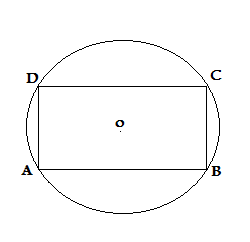a) 270°

b) 360°

c) 90°

d) 180°

Question 2: The height of an equilateral triangle is 15 cm. The area of the triangle is

a) 50√3 sq. cm.

b) 70√3 sq. cm.

c) 75√3 sq. cm.

d) 150√3 sq. cm.

Question 3: If the interior angles of a five-sided polygon are in the ratio of 2 : 3 : 3 : 5 : 5, then the measure of the smallest angle is

a) 20°

b) 30°

c) 60°

d) 90°

Question 4: If the lengths of the sides of a triangle are in the ratio 4 : 5 : 6 and the inradius of the triangle is 3 cm, then the altitude of the triangle corresponding to the largest side as base is :

a) 7.5 cm

b) 6 cm

c) 10cm

d) 8 cm

Question 5: The ratio of inradius and circumradius of a square is :

a) 1 : √2

b) √2 : √3

c) 1 : 3

d) 1 : 2

Question 6: AB and CD are two parallel chords of a circle such that AB = 10 cm and CD = 24 cm. If the chords are on the opposite sides of the centre and distance between them is 17 cm, then the radius of the circle is :

a) 11 cm

b) 12 cm

c) 13 cm

d) 10 cm

Question 7: A fraction becomes 9/11 , if 2 is added to both the numerator and the denominator. If 3 is added to both the numerator and the denominator it becomes 5/6 . What is the fraction ?

a) $\frac{7}{9}$

b) $\frac{3}{7}$

c) $\frac{5}{9}$

d) $\frac{7}{10}$

Question 8: If the circumradius of an equilateral triangle ABC be 8 cm, then the height of the triangle is

a) 16 cm

b) 6 cm

c) 8 cm

d) 12 cm

Question 9: Let ABC be an equilateral triangle and AX, BY, CZ be the altitudes. Then the right statement out of the four given responses is

a) AX = BY = CZ

b) AX BY = CZ

c) AX = BY # CZ

d) AX # BY # CZ

Question 10: Two supplementary angles are in the ratio 2 : 3. The angles are

a) 33°, 57°

b) 66°, 114°

c) 72°, 108°

d) 36°, 54°Sum of opposite angle of cyclic quadrilateral is 180° (D)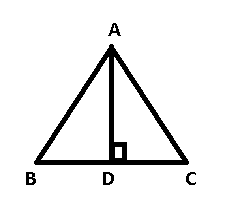AD = 15 cm and ABC is equilateral triangle

In $\triangle$ADC

=> $tan \angle ACD = \frac{AD}{DC}$

=> $tan 60 = \frac{15}{DC}$

=> DC = $\frac{15}{\sqrt{3}} = 5\sqrt{3}$

=> BC = 2*DC = $10\sqrt{3}$

Area of $\triangle$ ABC = $\frac{\sqrt{3}}{4} * side^2$

= $\frac{\sqrt{3}}{4} * (10\sqrt{3})^2$

= $75\sqrt{3} cm^2$

Let the angles of the pentagon be 2x,3x,3x,5x and 5x

Sum of angles of a pentagon = $(n-2) * 180$°

=> 2x+3x+3x+5x+5x = 540°

=> x = 30°

=> Smallest angle = 2*30 = 60°

Let the sides of triangle be $4x , 5x$ and $6x$

Inradius(r) = 3 cm and semi perimeter(s) = $\frac{4x+5x+6x}{2} = 7.5x$

=> Area of triangle = $r * s$ = $22.5x$

Let altitude be $h$

Also area = $\frac{1}{2} * 6x *h = 22.5x$

=> $h = \frac{22.5}{3} = 7.5$cm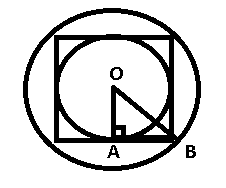Let the side of square be $a$

=> Inradius(OA) = $\frac{a}{2}$ = AB

In $\triangle$OAB

=> OB = $\sqrt{(OA)^2 + (AB)^2}$

=> OB = $\sqrt{(\frac{a}{2})^2 + (\frac{a}{2})^2}$

=> OB = $\sqrt{\frac{a^2}{4} + \frac{a^2}{4}} = \sqrt{\frac{a^2}{2}}$

=> OB = $\frac{a}{\sqrt{2}}$

To find : $\frac{OA}{OB}$

= $\frac{\frac{a}{2}}{\frac{a}{\sqrt{2}}}$

= $\frac{1}{\sqrt{2}}$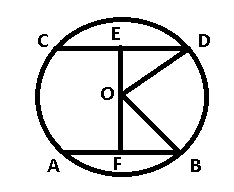Given : AB = 10 , CD = 24 and EF = 17 cm

To find : OB = OD = $r$ = ?

Solution : A line perpendicular to the chord from the centre of the circle bisects the chord.

=> $AF = FB = \frac{AB}{2} = \frac{10}{2} = 5$

Similarly, $CE = ED = 12$

Let OF = $x$ => OE = $(17-x)$

In right $\triangle$OFB

=> $(OB)^2 = (OF)^2 + (FB)^2$

=> $r^2 = x^2 + 25$

Now, in right $\triangle$OED

=> $(OD)^2 = (OE)^2 + (ED)^2$

=> $r^2 = (17-x)^2 + 144$

=> $x^2 + 25 = x^2 – 34x + 289 + 144$

=> $34x = 408$

=> $x = \frac{408}{34} = 12$

=> $r^2 = 12^2 + 25$

=> $r = \sqrt{169} = 13$ cm

Let the fraction be $\frac{x}{y}$

=> $\frac{x+2}{y+2} = \frac{9}{11}$

=> $11x + 22 = 9y + 18$

=> $11x – 9y + 4 = 0$

Also, $\frac{x+3}{y+3} = \frac{5}{6}$

=> $6x + 18 = 5y + 15$

=> $6x – 5y + 3 = 0$

Solving above equations, we get $x = 7$ and $y = 9$

=> Required fraction = $\frac{7}{9}$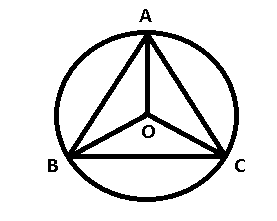Let ABC be the equilateral triangle and O be the circumcentre. AO extended meet BC at D.

In an equilateral triangle, the centroid, orthocentre, incentre and circumcentre, all lie on the same point, => the median and height are the same lines.

=> O is also the centroid of the triangle.

Since, the centroid divides the median in the ratio 2 : 1

It is given that OA = 8 cm

=> Height AD = $8 * \frac{3}{2}$ = 12 cm

In an equilateral $\triangle$ABC

$\angle$A = $\angle$B = $\angle$C = 60°

=> AB = BC = CA and hence AX = BY = CZ

Let the angles be $2x$ and $3x$

Since, the angles are supplementary

=> $2x + 3x = 180^{\circ}$

=> $x = \frac{180^{\circ}}{5}$

=> $x = 36^{\circ}$

=> Angles are 72° and 108°

we hope this Geometry Questions for SSC CGL is helpful to you.